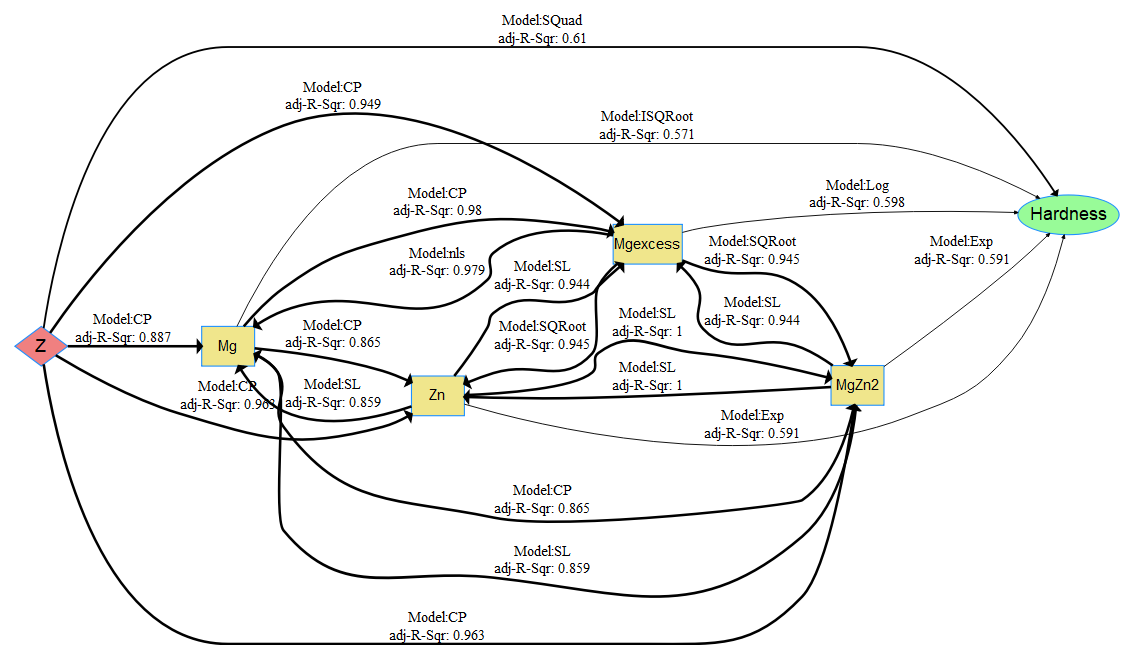# netSEM Modeling of the Aluminum Gradient Material for Metal’s Design

## Material Description

Functional graded materials (FGM) are a class of materials with engineered continuous compositional gradients through the plate thickness. This work applies the netSEM approach on an aluminum FGM, produced via sequential alloy casting using planar solidification, to quantify the < Processing | Microstructure | Performance > relationships. The material has a continuous gradient in zinc (Zn) and magnesium (Mg) concentrations through the plate thickness. This subsequently produces a gradient in strengthening mechanisms from a dominant precipitate-strengthened aluminum alloy (AA) (Zn-based AA-7055) to a dominant strain-hardenable aluminum alloy (Mg-based AA-5456). Therefore, the material is simultaneously strengthened via solid solution strengthening and precipitation strengthening.

## Data Collection

The data set comprises two aspects of the aluminum gradient material: composition (processing) and hardness (performance). Data was collected at six different x-locations (same composition along x) for each of the six different z-locations (different composition along z). For every hardness indent, two compositional measurements were taken, making the dataset a total of 72 observations. A Buehler 1600-4963 indenter with 300 gf load was used to measure Vickers hardness (HV) on polished samples as a function of position along the compositional gradient (z-direction). The compositional gradient was characterized using X-ray Energy Dispersive Spectroscopy (XEDS) in an FEI Nova NanoLab 200 at 10 KV beam energy. All parameters like working distance, accelerating voltage, current, dwell time, and dead time were held constant so that XEDS measurements from different samples/locations could be compared without bias.

## Data for netSEM

The aging experiments show MgZn2 ($$\eta$$) as a potential precipitate. The volume fraction of $$\eta$$ precipitates require a 2:1|Zn:Mg stoichiometry and therefore the volume fraction of precipitates are limited by the minimum of either the Zn/2 or Mg concentrations. For the compositional range of the FGM, this minimum is always Zn/2; therefore, $$\eta$$ concentration is proportional to the Zn(at%) concentration. This means that no Zn was retained in solid solution, and $$\eta$$ and Al(Mg) (i.e.,Mg retained in solid solution or Mg-excess) were used as variables for network model.

Resulting dataframe contains Position : Z as the main exogenous variable and Hardness as the endogenous variable (response). The other columns in the data set (Mg (at%), Zn (at%), MgZn2 ($$\eta$$), and Mg-excess) are variables representing the mechanistic variables in the netSEM analysis.

## Load data and run code to build netSEM

## Load the PET data set
data(metal)

## Run netSEM
ans <- netSEMm(metal, str = TRUE)

## Subset dataset with three cutoff
res <- subsetData(ans,cutoff=c(0.3,0.6,0.8))

## Plot the network model
plot(ans,res)

Network diagram for data
--------------------------
"Hardness" is the endogenous and all other variables are considered as exogenous.## Discussion

The network diagram shows a logarithmic relationship between Mg-excess and Hardness, and a exponential relationship between $$\eta$$ phase and Hardness. Visualization of the relationship between Mg-excess and Hardness indicate that functional forms (Log and Solid Solution(SS)) have similar shapes within ranges of the variables collected. The domain knowledge biases the selection of SS functional form for the network model betweeen Al(Mg) (or Mg-excess) and Hardness over the selection of the Log function, as it is consistent with the Mg concentration primarily contributing through solid-solution hardening. The Exp function with respect to the $$\eta$$ concentration could be consistent with diffusional mechanisms controlling the precipitate distribution and thus the strengthening behavior.

## Reference

Verma, A.K., French, R.H. & Carter, J.L.W. Integr Mater Manuf Innov (2017) 6: 279. https://doi.org/10.1007/s40192-017-0104-5

## Acknowledgment

The authors would like to acknowledge Alcoa/Arconic Alloy Technology Division for providing the material and Army Research Office Short Term Innovative Research program: W911NF-14-0549 for funding. The data and R-analytics code utilized for the paper can be found at https://cwru-msl.github.io/MRL17/.